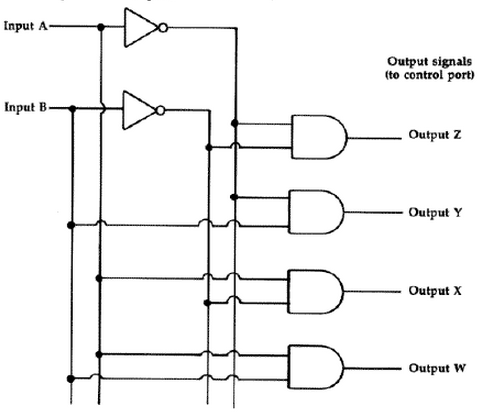# Why is Array Access Constant Time

By Xah Lee. Date: . Last updated: .

theory of the century: Array is constant random access time but list is not, because array is implemented with quantum bits.

in 20 years, i've not seen a programer who can explain why array has constant time access, but they all think they know. (actually, 1 person i've seen explained it)

Wikipedia's 4 thousands words on array, only has this handwaving: “They effectively exploit the addressing logic of computers.”

I have always wondered about this. You know that retrieving a indexed item of array takes O(1) time. Why is that? You have n things, and you want the kth item. It should be O(k) for kth item. It is impossible to get it with O(1).

The answer can't explained in software or algorithm. It really have to do with how the hardware is implemented. This article explains: [Why is Indexing Faster Than Binary Search By Yin Wang. At http://yinwang0.wordpress.com/2013/04/02/indexing/]

## Why is indexing faster than binary search

by Yin Wang,

We all know that indexing into an array takes only O(1) time, while searching for a number in a sorted array takes O(n) time with linear search, and O(log n) time with binary search. But why is indexing so fast? What is the secret sauce?

The reason is really about the nature of indexing — how it is implemented in a circuit. In order to illustrate this, let me show you an “addressing circuit”.Here, A and B are the two-bit address lines, they represent the indices: 00, 01, 10, 11. The output Z, Y, X and W are the selectors of the items in the array. Notice that an output selector is enabled only when both of the input lines of the corresponding AND gate is “1”.

Now, ignore the input B and just look at A. See how its signal flows through the direct wires and the inverters. We can make the following observations:

When A is “1”, then the AND gate of X and W will receive a “1” on one of their input ports, while the AND gate of Z and Y will receive a “0” on one of their input puts. On the other hand, if A is “0”, then the AND gate of X and W will receive a “0” on one of their input ports, while the AND gate of Z and Y will receive a “1” on one of their input puts.

From the above, I hope you have seen that the value of A partially selects half of the AND gates — it is either the set {X, W} or {Z, Y}. By “partially select”, I mean they are not fully selected, because we haven't taken B into account. At this point, I hope you have noticed that A is in fact doing one step of a “binary search”.

Now we do a similar thing, but this time focus on B and ignore A. You should see a similar thing: depending on the value of B, either we partially select {Y, W}, or we partially select {Z, X}. So we can also think of B as doing one step of a “binary search”.

Now, we see that A and B are each a step of a binary search, and it is interesting to see that B's selection will cut A's selection evenly, whether A is 0 or 1. We can say the same thing vice versa: A's selection will cut B's selection evenly, whether A is 0 or 1.

Also notice that the selection of A and B can happen at the same time. That means, when they work simultaneously, it takes just O(1) for a binary search through an array of length 4. If we generalize this circuit to N bits of input, then within O(1) time, we can do a binary search through an array of length 2N.

This explains why indexing an array is faster than binary search, because it is a parallel binary search where (log n) steps happen at the same time.

### speed of array vs list by the mouthing of hacker idiots

speed of array vs list, is one of the most misunderstood thing mouthed by hacker idiots. their speed properties, has nothing to do with math of algorithms, but hardware. more specifically, parallel nature of electronics of memory circuits.

i struggled to understand this for over 10 years. the question is this: why array has constant random access time. (but not list) ask a hacker programer, they'll drivel. (including text books) the real answer is, parallel electronic circuits.

this non-math/algorithm properties of speed in programing languages, is a huge problem. i think the problem is that we don't think of hardware esp in high level langs, such as python haskell. but ALL concrete algorithms must have a machine to work on.

when coding high level lang eg python haskell, we no think hardware, cuz the compiler to machine code is incomprehensible. a solution is for each lang to also specify a pseudo high level hardware, so any algorithmic properties of the lang's constructs r apparent to eyeball.

the entire scott meyers' talk, is a exhibit of the disconnect of programing lang and hardware.

it's funny. when coding python haskell etc, sequential algorithm in nature, but when you have myArray, then suddenly, bang, parallel computing is actually going on. This is all the misinfo and complexity began.

not to mention speculative execution, branch prediction, cache prefetchin etc. meltdown and spectre are 2 consequences of these non-math voodoo.